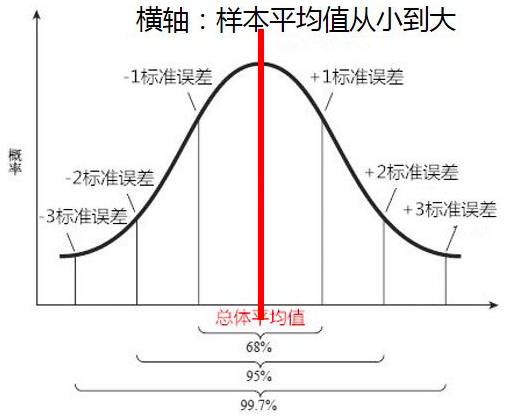# 小白都能看懂的95%置信区间

## 4.标准差(standard deviation)与标准误差(standard error)

$SE=\frac{s\left(样本标准差\right)}{\sqrt{n}}$

## 5.如何理解95%的置信区间

$X\sim N\left(\mu ,{\sigma }^{2}\right)$

$M=\frac{{X}_{1}+{X}_{2}+\cdots +{X}_{n}}{n}$

$M\sim N\left(\mu ,{\sigma }_{1}^{2}\right)$$P\left(\mu -1.96\frac{\sigma }{\sqrt{n}}

## 6.计算置信区间的套路

1.首先明确要求解的问题。比如我们的例子，就是想通过样本来估计全国人民身高的平均值。
2.求抽样样本的平均值与标准误差(standard error)。注意标准误差与标准差(standard deviation)不一样。
3.确定需要的置信水平。比如常用的95%的置信水平，这样可以保证样本的均值会落在总体平均值2个标准差得范围内。
4.查z表，求z值。
5.计算置信区间
a = 样本均值 - z*标准误差
b = 样本均值 + z*标准误差

$\overline{x}±z\frac{s}{\sqrt{n}}$

©️2019 CSDN 皮肤主题: 编程工作室 设计师: CSDN官方博客